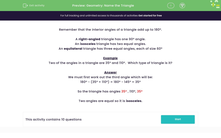# Name the Triangle

In this worksheet, students will work out the missing angle in a triangle in order to identify the type of triangle.Key stage:  KS 2

Curriculum topic:   Geometry: Properties of Shapes

Curriculum subtopic:   Compare Geometric Shapes

Popular topics:   Geometry worksheets

Difficulty level:#### Worksheet Overview

Remember that the interior angles of a triangle add up to 180º.

right-angled triangle has one 90º angle.

An isosceles triangle has two equal angles.

An equilateral triangle has three equal angles, each of size 60º

scalene triangle has no equal angles.Example

Two of the angles in a triangle are 35º and 110º.  Which type of triangle is it?

We must first work out the third angle which will be:

180º - (35º + 110º) = 180º - 145º = 35º

So the triangle has angles 35º , 110º, 35º

Two angles are equal, so it is isosceles.

Are you ready to have a go at this now?If you want to look at this introduction again during the activity, just click on the red help button that will appear on the screen.

### What is EdPlace?

We're your National Curriculum aligned online education content provider helping each child succeed in English, maths and science from year 1 to GCSE. With an EdPlace account you’ll be able to track and measure progress, helping each child achieve their best. We build confidence and attainment by personalising each child’s learning at a level that suits them.

Get started